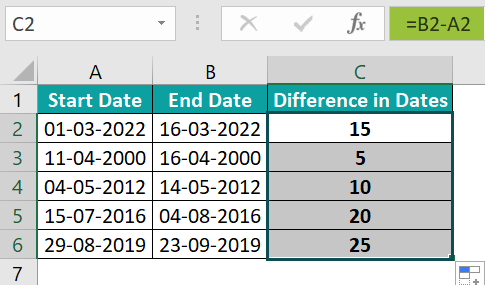# Date Range In ExcelArticle byExcelMojo Team## What Is Date Range In Excel?

The Date Range in Excel converts the date value to text in a specified format. It evaluates the range between two specified dates as per the pre-set numeric code.

The Excel Date Range is set to format the cell with the start and end date value as in date format and use the operation ‘+’ or ‘-’ to reflect the end date or Date Range.

For example, the image shows the start and end dates, and we will calculate the Date Range using the Ampersand (&) and the TEXT() function.

Select cell C2, enter the formula =TEXT(A2,“dd mmmm yyyy”)&“-”&TEXT(B2,“dd mmmm yyyy”), and press the “Enter” key.

The output is ‘10 January 2023 – 25 January 2023’, as shown above, i.e., the dates are combined because of the ampersand operator, separated with the “-” symbol.

###### Key Takeaways
• The Date Range in Excel converts a date value to its pre-set numeric code as per the performed calculations.
• If we want the result as Date/Text in a specified date format and not the numeric code, then we use the TEXT function.
• We can establish the Date Range between two dates using the Ampersand(&) operator and a separator “-”, “:”, etc.
• In a dataset, we can use the IF function to analyze whether the start or the end date is missing.
• We can build a sequence using simple arithmetic operations like adding 10 to a date and getting a new date, or finding the difference between dates for a single cell or multiple cells.
• The Excel Date Range checks the irregularities between the two dates by the range values.

### Syntax Of Date Range In Excel

The syntax of the Date Range formula is,

=TEXT(value, format_text) & “-” & TEXT(value, format_text)

The arguments of the Date Range formula are,

• Text() – The Excel TEXT Function converts the numeric values to their pre-set numeric code, or a numeric value into a text string.
• value: It is the numerical date value we must convert to text. It is a mandatory argument.
• format_text: It is the date format in excel we want to apply. It is a mandatory argument.
• ‘-’: It reflects the start and end dates in a Date Range format.

### How To Use Date Range In Excel?

We can use the Date Range as any other Excel function by typing the syntax in the target cell and selecting the cell references or cell values in place of the arguments. Let us see the same with an example.

Let us take an example with basic date ranges.

The following image shows the start date; we will calculate the start date and end date using the Date Range.

In the table, the data is,

• Column A contains the Start Date.
• Column B contains the End Date.

The steps to use the Date Range in excel are as follows:

1. Select cell B2, and enter the formula =A2+10.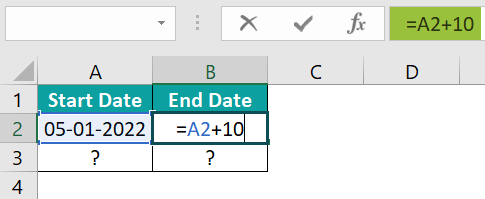2. Press the “Enter” key. The result is ‘15-01-2022’, as shown below.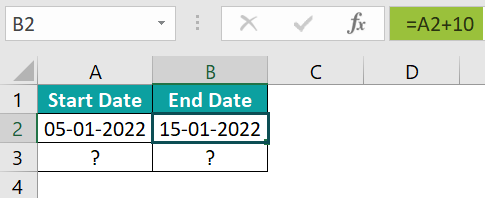3. Select cell A3, and enter the formula =B2+10.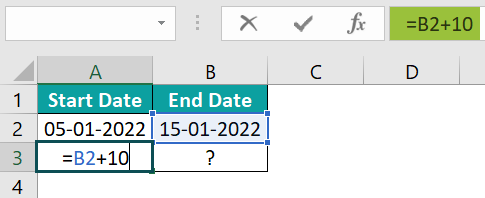4. Press the “Enter” key. The result is ‘25-01-2022’, as shown below.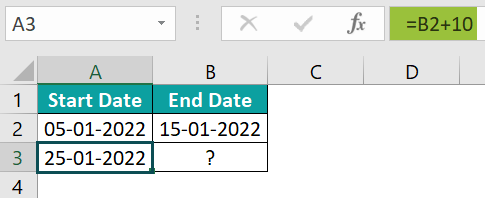5. Select cell B3, and enter the formula =A3+10.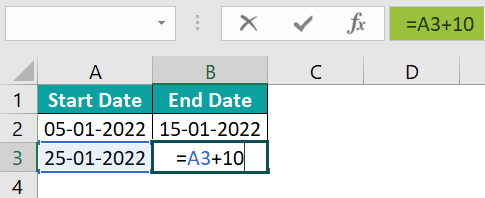6. Press the “Enter” key. The result is ‘04-02-2022’, as shown below.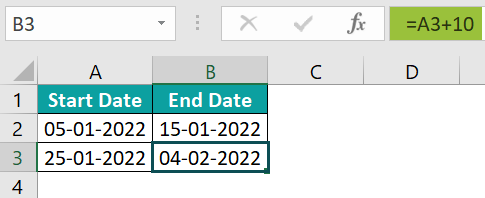7. Now, select cell range A2:B3, and drag to cells A6:B6 using the fill handle. The sequence is copied as shown below.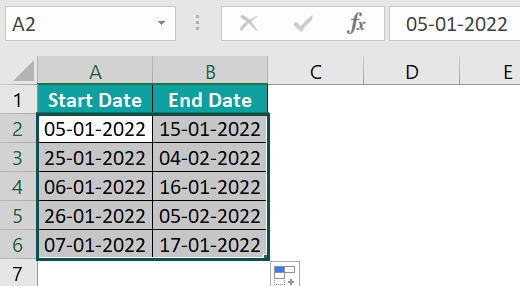### Examples

We will consider some advanced scenarios using the Date Range in Excel examples.

#### Example #1 – Creating Date Sequence

The following image shows the start date. We will calculate the start and end dates using the Creating Date Sequence.

In the table, the data is,

• Column A contains the Start Date.
• Column B contains the End Date.

The procedure to calculate the Date Range is,

Select cell range A2:B3, and drag the sequence, using the fill handle, to the rest of the cells, i.e., to A11:B11.

The output is shown below.

#### Example #2 – Date Range in a Single Cell

The following image shows the start date and end date, and we will calculate the Date Range in a single cell using the TEXT function.

In the table, the data is,

• Column A contains the Start Date.
• Column B contains the End Date.
• Column C displays the Date Range.

The steps to calculate the Date Range are,

• Step 1: Select cell C2, and enter the formula =TEXT(A2,“dd mmmm yyyy”)&“-”&TEXT(B2,“dd mmmm yyyy”).
• Step 2: Press the “Enter” key. The below image shows the output as ‘01 March 2023–16 March 2023’.

#### Example #3 – Date Range in Multiples Cells

The following image shows the start date and end date, and we will calculate the Date Range in multiple cells using the TEXT function.

In the table, the data is,

• Column A contains the Start Date.
• Column B contains the End Date.
• Column C displays the Date Range.

The steps to calculate the Date Range are as follows:

• Step 1: Select cell C2, and enter the formula =TEXT(A2,“dd mmmm yyyy”)&“-”&TEXT(B2,“dd mmmm yyyy”).
• Step 2: Press the “Enter” key. The result is ‘01 March 2023 – 16 March 2023’, as shown below.
• Step 3: Drag the formula from cell C3 to C6 using the fill handle. The output is shown below.

### Important Things To Note

• Adding a number to a date can formulate a date change.
• Date Range helps to bridge the gaps between the two Date Ranges.
• When we enter the separator we must use double-quotes, else we will get an error.
• We can find the difference between number of days of two dates using the operator ‘-’.

1. What is the use of Date Range in Excel?

The Excel Date Range helps to perform calculations on dates. It also calculates the range within two specified dates. The feature converts a date value to text in the required format.

2. What is the formula for calculating the Range of Dates in Excel?

To calculate the Date Range, we use 2 features, the ampersand (&) operator and the Text() function =Text(value, format text).

The combined syntax is =TEXT(value, format_text)& “ – ” & TEXT(value, format_text)

3. How to calculate the difference between two Dates in Excel?

The following image shows the start and end dates, and we will calculate the difference between the two Dates in Excel.

In the table, the data is,
• Column A contains the Start Date.
• Column B contains the End Date.
• Column C displays the Difference in Date.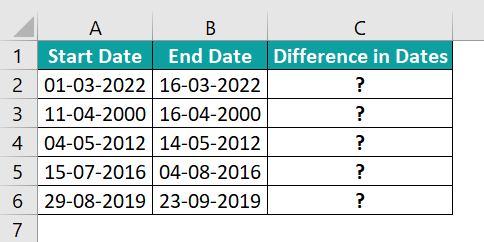The steps to calculate the difference in Date Range are as follows:

Step 1: Select cell C2, and enter the formula =B2-A2.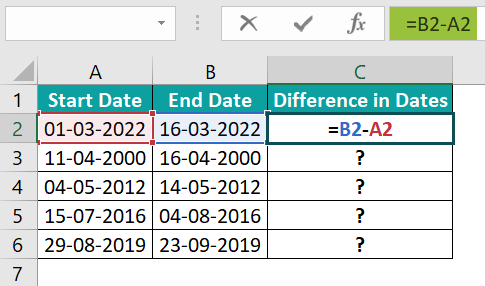Step 2: Press the “Enter” key. The difference result is ‘15’, as shown below.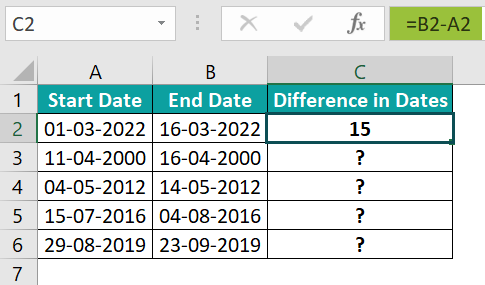Step 3: Drag the formula from cell C2 to C6 using the fill handle. The output is shown below.